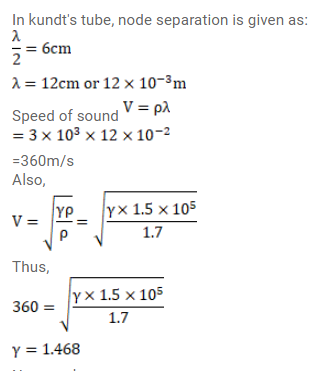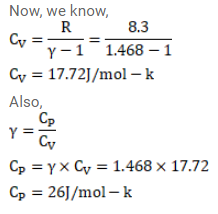# An ideal gas having density`
Question:

An ideal gas having density $1.7 \times 10^{-3} \mathrm{~g} / \mathrm{cm}^{3}$ at a pressure $1.5 \times 10^{5} \mathrm{~Pa}$ is filled in a Kundt's tube. When the gas is resonated at a frequency of $3.0 \mathrm{kHz}$, nodes are formed at a separation of $6.0 \mathrm{~cm}$. Calculate the molar heat capacities $C_{p}$ and $C_{v}$ of the gas.

Solution: